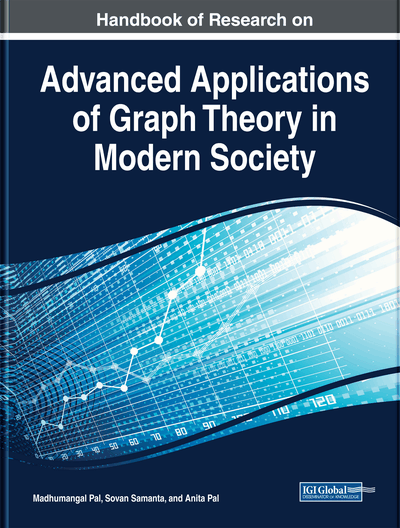# Graph Indices

V. R. Kulli (Gulbarga University, India)
DOI: 10.4018/978-1-5225-9380-5.ch003

## Abstract

A molecular graph is a finite simple graph representing the carbon-atom skeleton of an organic molecule of a hydrocarbon. Studying molecular graphs is a constant focus in chemical graph theory: an effort to better understand molecular structure. Many types of graph indices such as degree-based graph indices, distance-based graph indices, and counting-related graph indices have been explored recently. Among degree-based graph indices, Zagreb indices are the oldest and studied well. In the last few years, many new graph indices were proposed. The present survey of these graph indices outlines their mathematical properties and also provides an exhaustive bibliography.
Chapter Preview
Top

## Zagreb Indices

Let G be a simple graph with vertex set V(G) and edge set E(G). Let |V(G)|= n and |E(G)| = m. The edge connecting the vertices u and v will be denoted by uv. The degree dG(u) of u is the number of vertices adjacent to u.

A graph invariant is a function on a graph which is not depending on the labeling of its vertices. Such quantity is called a topological index or molecular descriptor or graph index. Graph indices play a significant role in Theoretical Chemistry, especially in, structure property relationships and structure activity relationships (Gutman, 2013, 1986; Todeschini & Consonni, 2009). In Chemical Science, graph indices have been found to be useful in chemical documentation, isomer discrimination and pharmaceutical drug design. There has been considerable interest in the general problem of determining graph indices. Wiener index (Wiener, 1947) is the best-known index and it is defined as the sum of the distances between all pairs of vertices of a graph G

This index is used for the calculation of the boiling points of alkenes. In Gutman and Trinajstić (1972), Gutman and Trinajstić (1972) introduced the first and second Zagreb indices, defined as

The first Zagreb index is defined as (Nikolic et al., 2003).

Many properties and chemical applications of these indices can be found in Caparossi, Hansen, and Vukicevic (2010), Das and Gutman (2004), Das, Gutman, and Horoldagya (2012), Das, Gutman,a nd Zhou (2009), Das, Xu, and Gutman (2013), Doslic et al. (2011), Fath-Tabar (2011), Gutman and Das (2004), Hosamani and Gutman (2014), Ilic and Stevanovic (2011), Khalifeh, Yousefi-Azari, and Ashrafi (2009), Liu and Yoi (2011), Ranjini, Lokesha, and Cangul (2011), Reti (2012), Trinajstić et al. (2010), Xu et al. (2015), and Zhou (2004, 2007). Several results on Zagreb indices were established and many modifications of Zagreb indices were introduced.

Shirdel et al. proposed the first hyper Zagreb index (Shirdel, Rezapour & Sayadi, 2013)

Some properties of the first hyper Zagreb index were obtained (Basavanagoud & Patil, 2016; Falahati, Nezhad & Azari, 2016; Gao, Jamil & Farahani, 2017; Gutman, 2017; Luo, 2016; Pattabiraman & Vijayaragayan, 2017).

## Complete Chapter List

Search this Book:
Reset